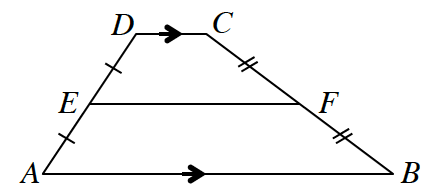### Home > INT1 > Chapter 7 > Lesson 7.2.2 > Problem7-99

7-99.

In this lesson, you discovered that the midsegment of a triangle is not only parallel to one of the sides, but also half that side’s length. But what about the midsegment of a trapezoid?

The diagram at right shows a midsegment of a trapezoid. That is, $\overline{EF}$ is a midsegment because points $E$ and $F$ are both midpoints of the non-base sides of trapezoid $ABCD$.

1. If $A(0, 0)$, $B(9, 0)$, $C(5, 6)$, and $D(2, 6)$, find the coordinates of points $E$ and $F$. Then compare the lengths of the bases ($\overline{AB}$ and $\overline{CD}$) with the length of the midsegment $\overline{EF}$. What is the relationship among the segments?

How do you find the lengths from coordinates?
Think of how the answers could be related.

It seems to be the average of $AB$ and $CD$.

2. Verify that the relationship you observed in part (a) holds if $A(–4, 0)$, $B(2, 0)$, $C(0, 2)$, and $D(–2, 2)$.

Use the same method you used to solve part (a).

3. Write a conjecture about the midsegment of a trapezoid.

The midsegment of a trapezoid is parallel to the bases and has a length that is the average of the lengths of the bases.Use the eTool below to help solve the problem.
Click the link at the right to view full version of the eTool: Int1 7-99 HW eTool.